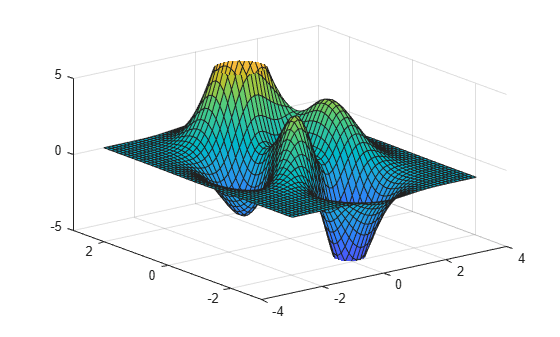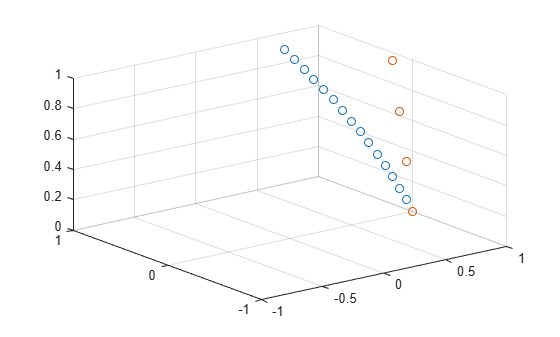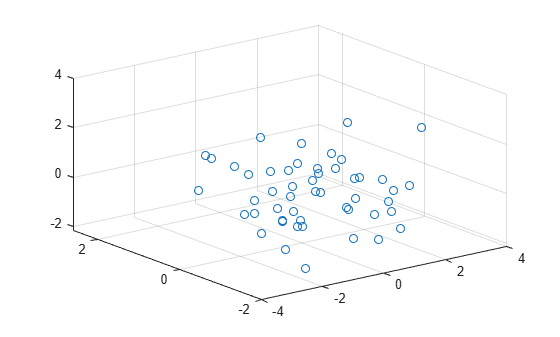Documentation

zlim

Set or query z-axis limits

Description

example

zlim(limits) sets the z-axis limits for the current axes. Specify limits as a two-element vector of the form [zmin zmax], where zmax is greater than zmin.

example

zl = zlim returns the current limits as a two-element vector.

zlim auto sets an automatic mode, enabling the axes to determine the z-axis limits. The limits span the range of the plotted data. Use this option if you change the limits and then want to set them back to the default values. This command sets the ZLimMode property for the axes to 'auto'.

example

zlim manual sets a manual mode, freezing the limits at the current values. Use this option if you want to retain the current limits when adding new data to the axes using the hold on command. This command sets the ZLimMode property for the axes to 'manual'.

m = zlim('mode') returns the current z-axis limits mode, which is either 'auto' or 'manual'. By default, the mode is automatic unless you specify limits or set the mode to manual.

example

___ = zlim(ax,___) uses the axes specified by ax instead of the current axes. Specify ax as the first input argument for any of the previous syntaxes. You can include an output argument if the original syntax supports an output argument. Use single quotes around the mode inputs, for example, zlim(ax,'auto').

Examples

collapse all

Plot a surface and set the z-axis limits to range from -5 to 5.

[X,Y,Z] = peaks;
surf(X,Y,Z);
zlim([-5 5])Create a mesh surface plot and show only z values greater than 0. Specify the minimum z-axis limit as 0 and let MATLAB choose the maximum limit.

[X,Y,Z] = peaks;
mesh(X,Y,Z)
zlim([0 inf])Starting in R2019b, you can display a tiling of plots using the tiledlayout and nexttile functions. Call the tiledlayout function to create a 2-by-1 tiled chart layout. Call the nexttile function to create the axes objects ax1 and ax2. Plot data into each of the axes. Then set the z-axis limits for the bottom plot by specifying ax2 as the first input argument to zlim.

[X,Y,Z] = peaks;
tiledlayout(2,1)
ax1 = nexttile;
surf(X,Y,Z)

ax2 = nexttile;
surf(X,Y,Z)
zlim(ax2,[-5 5])Use manual mode to maintain the current z-axis limits when you add more plots to the axes.

First, create a 3-D scatter plot.

theta = linspace(0,2*pi);
X = cos(theta);
Y = sin(theta);
Z = theta;
scatter3(X,Y,Z)Set the z-axis limits mode to manual so that the limits to not change. Use hold on to add a second plot to the axes.

zlim manual
hold on
Znew = 5*theta;
scatter3(X,Y,Znew)
hold offThe z-axis limits do not update to incorporate the new plot.

Switch back to automatically updated limits by setting the mode to automatic.

zlim autoCreate a 3-D scatter plot of random data. Return the values of the z-axis limits.

x = randn(50,1);
y = randn(50,1);
z = randn(50,1);
scatter3(x,y,z)zl = zlim
zl = 1×2

-3     3

Input Arguments

collapse all

Minimum and maximum limits, specified as a two-element vector of the form [zmin zmax], where zmax is greater than zmin. You can specify the limits as numeric, categorical, datetime, or duration values. However, the type of values that you specify must match the type of values along the z-axis.

You can specify both limits, or specify one limit and let MATLAB® automatically calculate the other. For an automatically calculated minimum or maximum limit, use -inf or inf, respectively.

Example: zlim([0 1])

Example: zlim([-inf 1])

Example: zlim([0 inf])

Data Types: single | double | int8 | int16 | int32 | int64 | uint8 | uint16 | uint32 | uint64 | categorical | datetime | duration

Axes object. If you do not specify an Axes object, then zlim sets the limits for the current axes (gca).

Output Arguments

collapse all

Current limits, returned as a two-element vector of the form [zmin zmax].

Querying the limits returns the ZLim property value for the corresponding Axes object.

Current limits mode, returned as one of these values:

• 'auto' — Automatically determine the limits.

• 'manual' — Use manually specified limits that do not update to reflect changes in the data.

Querying the z-axis limits mode returns ZLimMode property value for the corresponding Axes object.

Algorithms

The zlim function sets and queries several axes properties related to the z-axis limits.

• ZLim — Property that stores the z-axis limits.

• ZLimMode — Property that stores the z-axis limits mode. When you set the z-axis limits, this property changes to 'manual'.